DraftKings Super Bowl Odds
+600
6 to 1Bills
11.3% implied probability

+700
7 to 1Buccaneers
9.9% implied probability

+1000
10 to 1Chiefs
7.2% implied probability

+1000
10 to 1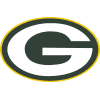Packers
7.2% implied probability

+1000
10 to 1Rams
7.2% implied probability

+1400
14 to 1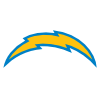Chargers
5.3% implied probability

+1600
16 to 1Broncos
4.7% implied probability

+1600
16 to 1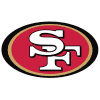49ers
4.7% implied probability

+1800
18 to 1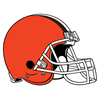Browns
4.2% implied probability

+1800
18 to 1Cowboys
4.2% implied probability

+2000
20 to 1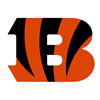Bengals
3.8% implied probability

+2200
22 to 1Ravens
3.5% implied probability

+2200
22 to 1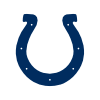Colts
3.5% implied probability

+2500
25 to 1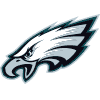Eagles
3.1% implied probability

+3500
35 to 1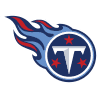Titans
2.2% implied probability

+3500
35 to 1Cardinals
2.2% implied probability

+4000
40 to 1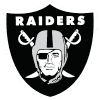Raiders
1.9% implied probability

+4000
40 to 1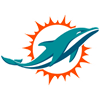Dolphins
1.9% implied probability

+5000
50 to 1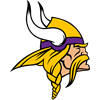Vikings
1.6% implied probability

+5000
50 to 1Patriots
1.6% implied probability

+5000
50 to 1Saints
1.6% implied probability

+6000
60 to 1Commanders
1.3% implied probability

+8000
80 to 1Steelers
1.0% implied probability

+10000
100 to 1Panthers
0.8% implied probability

+13000
130 to 1Jaguars
0.6% implied probability

+13000
130 to 1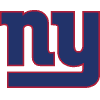Giants
0.6% implied probability

+13000
130 to 1Jets
0.6% implied probability

+13000
130 to 1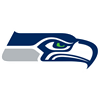Seahawks
0.6% implied probability

+15000
150 to 1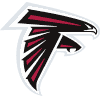Falcons
0.5% implied probability

+15000
150 to 1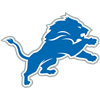Lions
0.5% implied probability

+15000
150 to 1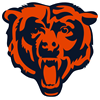Bears
0.5% implied probability

+25000
250 to 1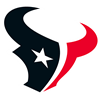Texans
0.3% implied probability